# Difference between revisions of "Dipole moment"

The electric dipole moment is a measure of polarity. It is the second term in a multipole expansion of a field (the first one being the monopole, or Coulombic, term.) Its definition, for a system of point charges, is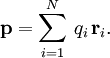$\mathbf{p} = \sum_{i=1}^N \, q_i \, \mathbf{r}_i.$
For molecular systems, it is customarily given in units of Debyes, after the physicist Peter J. W. Debye. In SI units,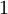$1$D equals approximately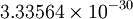$3.33564\times 10^{-30}$ Coulomb-meter (exactly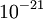$10^{-21}$C m$^2$/s divided by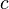$c$, the speed of light in vacuum). Conversely, 1 C m =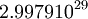$2.9979 10^{29}$D.